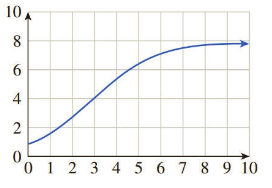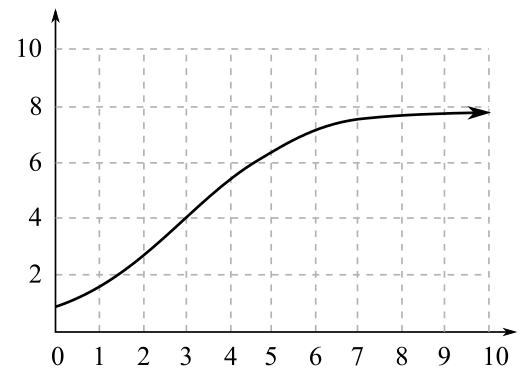Chapter 2.4, Problem 12EFinite Mathematics and Applied Cal...

7th Edition
Stefan Waner + 1 other
ISBN: 9781337274203

Solutions

Chapter
SectionFinite Mathematics and Applied Cal...

7th Edition
Stefan Waner + 1 other
ISBN: 9781337274203
Textbook Problem

In Exercises 11-16, choose the logistic function that best approximates the given curve.(A) f ( x ) = 8 1 + 7 ( 2 ) − x (B) f ( x ) = 8 1 + 3 ( 2 ) − x (C) f ( x ) = 6 1 + 11 ( 5 ) − x

To determine

The correct logistic function f

from the given choices for the following curve,(A). f(x)=81+7(2)x

(B). f(x)=81+3(2)x

(C). f(x)=61+11(5)x

Explanation

Given Information:

The provided curve is,

The given choices for the correct logistic function are,

(A). f(x)=81+7(2)x

(B). f(x)=81+3(2)x

(C). f(x)=61+11(5)x

The form of the logistic function is:

f(x)=N1+A(bx)

Where constants N,A

and b

are non-zero (A,bare positive and b1).

There are certain properties of the curve represented by the logistic curve, y=N1+A(bx)

1.

The graph of the function formed is S-shaped which is formed by sandwiching between the horizontal lines y=0 and y=N, which means the limiting value of the function is N.

2.

If b>1, graph rises, if b<1, the graph falls.

Now, the provided curve is,

It is clear from the curve that the function f

has limiting value 8, which means the value of constant N=8

Still sussing out bartleby?

Check out a sample textbook solution.

See a sample solution

The Solution to Your Study Problems

Bartleby provides explanations to thousands of textbook problems written by our experts, many with advanced degrees!

Get Started

Find the limit or show that it does not exist. limxx+3x24x1

Single Variable Calculus: Early Transcendentals, Volume I

In Exercises 1-6, simplify the expression. 27+12

Calculus: An Applied Approach (MindTap Course List)

In Exercises 4562, find the values of x that satisfy the inequality (inequalities). 49. 6 x 2 4

Applied Calculus for the Managerial, Life, and Social Sciences: A Brief Approach

In problems 37-44, perform the indicated operations and simplify. 38.

Mathematical Applications for the Management, Life, and Social Sciences

True or False: is a convergent series.

Study Guide for Stewart's Single Variable Calculus: Early Transcendentals, 8th